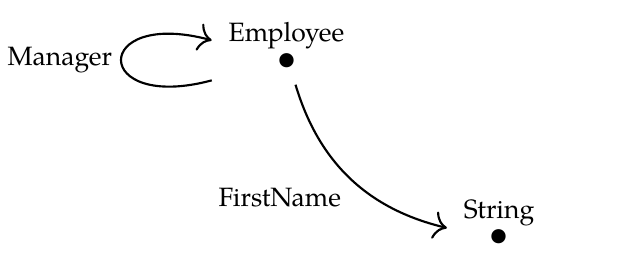Sophie wrote:

> In the example of transforming databases from [Lecture 41](https://forum.azimuthproject.org/discussion/2230/lecture-41-chapter-3-composing-functors), the functor that ignores departmental data is full and faithful but not essentially surjective. So it "forgets at most properties". I can't figure out how to think of departmental data as a property but maybe I am trying to take the analogy too far?

You can never take this analogy - or more precisely, this concept of 'stuff, structure and properties' - too far. But I'm a bit confused.

Let me remember what we were talking about. We created a category \$$\mathcal{C}\$$ by taking the free category on this graph:and a category \$$\mathcal{D}\$$ by taking the free category on this graph:We got an obvious 'inclusion' functor \$$G : \mathcal{D} \to \mathcal{C}\$$.

It sounds like you're asking whether \$$G\$$ is full, faithful and/or essentially surjective, and asking what this means in terms of stuff, structure and properties. That's fun to think about.

But here's another fun thing to think about! "Composing with \$$G\$$" turns databases built on the schema \$$\mathcal{C}\$$ into databases built on the schema \$$\mathcal{D}\$$. In other words, we have a functor

$\textrm{ composing with } G \; : \mathbf{Set}^\mathcal{C} \to \mathbf{Set}^\mathcal{D}$

We can also ask if _this_ functor is full, faithful and/or essentially surjective, and what _this_ means in terms of stuff, structure and properties.

I think I was confused, at first, about what you were thinking about. Now I think it's really the first, since \$$G\$$ is indeed full, faithful but not essentially surjective.

I have more to say, but not now, since it's Brandon Coya's graduation and I have to go put a hood on him!